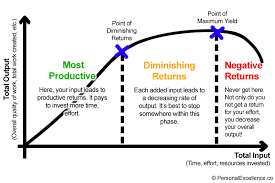# Law of diminishing returns & the three stages of production

The law of diminishing returns describes the relationship between output and the variable input when other inputs are held constant.

Definition: If increasing amounts of one input are added to a production process while all other inputs are held constant, the amount of output added per unit of variable input will eventually decrease. It is also known as law of diminishing productivity or the law of variable proportions.Application of the law of diminishing returns to the production concept can result in a production function of classical type. It displays increasing marginal returns first and then decreasing marginal returns.

### Three stages of production

The classical production function can be divided into three regions or stages, each being important from the standpoint of efficient resources use.

Stage-I occurs when marginal physical product (MPP) > average physical product (APP). APP  is increasing throughout this stage, indicating that the average rate at which X is transformed into Y, increases until APP reaches its maximum at the end of Stage-I.

Stage-II occurs when MPP is decreasing and is less than APP but greater than zero. The physical efficiency of the variable input reaches a peak at the beginning of Stage–II. On the other hand physical efficiency of fixed input is greatest at the end of Stage-II. This is because the number of fixed input is constant and therefore the output/ unit of fixed input must be the largest when the total output from the production process is maximum.

Stage-III occurs when MPP is negative. Stage III occurs when excessive quantities of variable input are combined with the fixed input, so much, that total physical product (TPP) begins to decrease.

Economic recommendations & production function analysis: Production function knowledge and the input and output prices information can be used to know the most profitable input and output levels. However, even when price information is not available, some recommendations about the input use can be made from the production function itself.

1. If the product has any value at all, input use once begun, should be continued until Stage

–II is reached. That is because physical efficiency of variable resources, measured by APP, increases throughout stage –I.

1. Even if input is free, it will not be used in stage III. Maximum output occurs when Stage II closes. It is of no use applying variable input when TPP starts coming
2. Stage II defines the area of economic relevance. Variable input use must be somewhere in stage-II, but exact input amount can be determined when choice indicators (input & output prices) are

### A.   Relationship between TPP & MPP

1. Since MPP is a measure of rate of change, therefore
• when TPP is increasing, MPP will be +ve,
• when TPP is constant MPP will be zero,
• when TPP decreases, MPP will be –ve.
1. So long MPP moves upward, TPP increases at an increasing
1. When MPP remains constant, TPP increases at a constant
1. When MPP starts declining, TPP increases at a decreasing
1. When MPP is zero, TPP will be at

### B.   Relationship between MPP & APP

1. When MPP is increasing, APP is also increasing. So long as MPP is above APP, the APP keeps
2. When MPP curve goes below APP curve, APP starts declining, that is, when AP is decreasing the MP is always less than
3. When MP = AP, AP will be at maximum. Here MP curve must intersect AP curve from above at its highest

So when          MP > AP            AP↑

MP < AP            AP↓

MP = AP         AP is at maximum.

Elasticity of production: The elasticity of prodLaw of diminishing returns & the three stages of production AssignmentLaw of diminishing returns & the three stages of production AssignmentLaw of diminishing returns & the three stages of production AssignmentLaw of diminishing returns & the three stages of production Assignmentuction is a concept that measures the degree of responsiveness between output and input. It is independent of the units of measurement.

E = % change in output

p            % change in input

E   =  DY / Y   DY / DX  MPP

p          DX / X         Y / X         APP

Ep > 1 in Stage I

0 £ E p £ 1     stage II

is based on exact MPPs

Ep is negative        stage III

The point of diminishing returns can be defined to occur when MPP =APP that is Ep= 1 (lower boundary of stage II) & this is the minimum amount of variable input that will be used & it occurs when the efficiency of variable input is at its maximum. At the other end, MPP is zero, therefore Ep= 0. Thus the relevant production zone is when O ≤ Ep ≤ 1.

The optimum level of variable input is given by:

### ΔX1.PX1  =  ΔY. Py or    ΔY/ ΔX1. =PX1/ Py

Marginal cost = marginal revenue.

## STEM Elearning

We at FAWE have built this platform to aid learners, trainers and mentors get practical help with content, an interactive platform and tools to power their teaching and learning of STEM subjects, more

#### How to find your voice as a woman in Africa

© FAWE, Powered by: Yaaka DN.